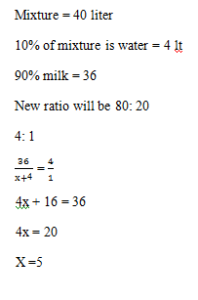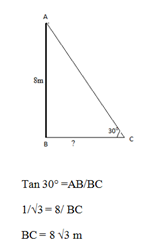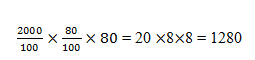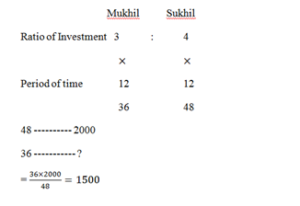# RRB ALP 2018 Practice Test Papers | Arithmetic Questions (Day-64)

Dear Aspirants, Here we have given the Important RRB ALP & Technicians Exam 2018 Practice Test Papers. Candidates those who are preparing for RRB ALP 2018 can practice these Arithmetic Questions to get more confidence to Crack RRB 2018 Examination.

[WpProQuiz 2879]

Click “Start Quiz” to attend these Questions and view Solutions

1) 40 liters of a mixture of milk and water contains 10% of water, the water to be added to make the water content 20% in the new mixture. Find how many liters water will be added?

a) 10

b) 5

c) 8

d) 9

2) A ladder is resisting a wall of height  8 m. If the ladder is inclined with the ground at an angle of 30°, then the distance of the foot of the ladder from the wall is

a) 8/√3

b) 8√3

c) 4

d) 2√3

3) Find the expression value of 85÷17 ×285÷95 =?

a) 5

b) 10

c) 15

d) 20

4) An Article Marked for sale of Rs.2000, What is the selling price, after selling two successive discounts of 20%?

a) 1720

b) 1520

c) 1280

d) 1260

1. The value of √ (√64 × ( 481))

a) 7

b) 8

c) 12

d) 6

1. A train travelling at a speed of 15 m/sec crosses a 300 m long platform in 25s. Find the length of the train.

a) 150m

b) 300m

c) 75 m

d)  60m

7) Rohit can build a Compound wall in 15 days. Mohit can do this same job in 10 days. How long the compound wall can complete if they together do this work?

a) 8 days

b) 10 days

c) 6 days

d) 9 days

8) Mukhil and Sukhil entered into the business with the investment ratio of 3:4. At the end of 1 year Sukhil earned a profit of Rs.2000. Then what is the investment amount of Mukhil?

a) 1000

b) 1700

c) 1500

d) 2000

1. What is the Value of 50% of 500+ ¾ of 200?

a) 50

b) 100

c) 200

d) 400

HCF and LCM of two numbers is 6 and 72 respectively, One Number is ¾ of another number. Find the smallest number?

a) 20

b) 22

c) 18

d) 1685÷17 ×285÷95 = 5×3 = 15√ (√64 × (√ 4√81)) = √(8×2×9 )= √144 = 12

Speed of train = 15mps

Time = 15 s

Length = T×S = 15×25 = 375

Relative Distance = Length of Train + Length of Platform

375 = x+ 300

Length of Train = 75m

Rohit = 1/15 days

Mohit = 1/10 days

Rohit + mohit = 1/15 + 1/10 = (2+3)/30 = 5/30 = 1/6

They together can finish the Compound wall in 6 days50% of 500+ ¾ of 200 = 250+ 150 =400

First number × second number = HCF × LCM

(4x)×(3x)  = 72×6

12x2 = 72×6

X = 6

Smallest number = 6×3 = 18

RRB ALP 2018 – “All in One” Study Materials and Practice Sets